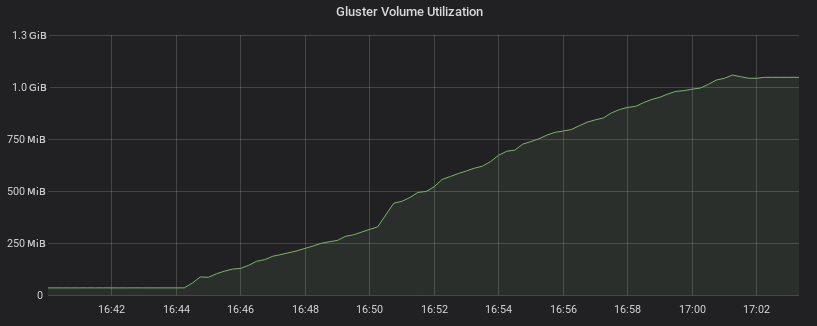# Monitoring GlusterFS - Volume Utilization

This blog explains the approaches to monitor Gluster Volume utilization using Prometheus.Gluster Volume Utilization visualized using Grafana

To get the Gluster Volume utilization, easy way is to use mount and df.

```mkdir /mnt/gv1
mount -t glusterfs localhost:gv1 /mnt/gv1
df /mnt/gv1
```

Alternatively we can use “libgfapi”(For example glusterdf tool uses “libgfapi”)

## Exporter from all nodes exports volume utilization

If Volume utilization is collected from each node then all the volumes needs to be mounted in all nodes and exporters will export duplicate data from all nodes.

```gluster_volume_utilization{instance="node1.example.com:8080",volname="gv1"} 790425600
```

If exported data is like above then add the following rule to Prometheus configuration so that duplicate data will be eliminated in the visualization.

```groups:
- name: gluster_volume_utilization
rules:
- record: gluster:volume_utilization_total:sum
expr: max(gluster_volume_utilization) by (volname)
```

## Exporter from one node exports volume utilization

If Volume utilization is collected from one leader node then that node will be overloaded by the mount processes if we have more number of volumes.

```leader_node = online_peers().sorted().first()
if (leader_node.id == local_node.id) {
export_volume_utilization()
}
```

In this approach, no aggregation rules required at Prometheus side but failover of a node needs to be handled to export the metrics if current leader goes down.

## Exporter from all nodes exports Brick utilization

Both the approaches mentioned above are inefficient unless we implement a common mount which provides all volumes utilization or glusterd aggregates the brick sizes and provides the Volume output.

With this approach export the brick utilization directly from each node and aggregating at the Prometheus server.

For example, let us consider a "2x3" Volume with bricks b1 to b6. If each node exporters publishes local bricks utilization, total volume utilization can be found using,

```subvol1_utilizaton = max(b1_utilization, b2_utilization, b3_utilization)
subvol2_utilizaton = max(b4_utilization, b5_utilization, b6_utilization)
volume_utilization = sum(subvol1_utilization, subvol2_utilization)
```

This formula works great for replicate volume, but in case of disperse Volume aggregated value will give wrong value, So multiply each brick's utilization with number of data bricks.

```subvol_size = number_of_data_bricks * per_brick_utilization
```

To accommodate this, exporter needs to be modified to export effective utilization along with raw utilization.

```subvol_utilization = df(brick_path).used
if (disperse_volume)
effective_subvol_utilization = brick_utilization * number_of_data_bricks
else
effective_subvol_utilization = brick_utilization
```

With the above two exported values, both Volume and brick utilization can be monitored without mounting the Gluster volume.

Example: Exported values for Volume gv1(Replica 3 volume)

```gluster_subvol_capacity_used_bytes{instance="node1.example.com:8080",job="gluster",path="/exports/bricks/gv1/s1/brick1/brick",subvolume="s1",volume="gv1"} 790425600
gluster_subvol_capacity_used_bytes{instance="node2.example.com:8080",job="gluster",path="/exports/bricks/gv1/s1/brick2/brick",subvolume="s1",volume="gv1"} 788611072
gluster_subvol_capacity_used_bytes{instance="node3.example.com:8080",job="gluster",path="/exports/bricks/gv1/s1/brick3/brick",subvolume="s1",volume="gv1"} 790175744
```

Add the following rule in the Prometheus configuration file to record the Volume utilization.

```---
- name: gluster_volume_utilization
rules:
- record: gluster:volume_capacity_used_bytes_total:sum
expr: >
sum(max(gluster_subvol_capacity_used_bytes)
by (volume, subvolume)) by (volume)
```

If one or more bricks of a sub volume goes down, it still exports correct Volume utilization if at least one brick is available. If all bricks of a sub volume goes down, then total Volume utilization will not include that sub volume utilization data. This is known limitation with all the approaches since the Volume itself is not fully available.

## Conclusion

Last approach provides same accuracy more efficiently(No Gluster mounts used) compared to other two alternatives.

Let me know your thoughts